# A 15.0-L tank is filled with helium gas at a pressure of1.00 x 102 atm. How many balloons (each 2.00 L) can beinflated to a pressure of 1.00 atm, assuming that the temperatureremains constant and that the tank cannot beemptied below 1.00 atm?

Question
4 views

A 15.0-L tank is filled with helium gas at a pressure of
1.00 x 102 atm. How many balloons (each 2.00 L) can be
inflated to a pressure of 1.00 atm, assuming that the temperature
remains constant and that the tank cannot be
emptied below 1.00 atm?

check_circle

Given data: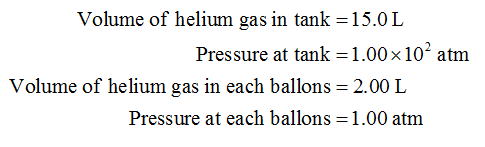Calcuklations:

The number of moles of helium is calculated by the formula shown below.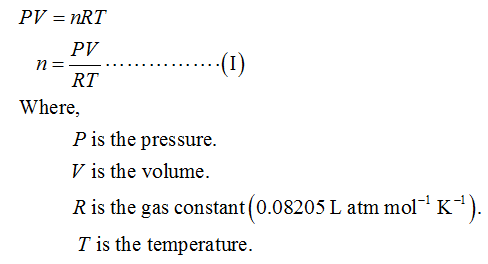Substitute the volume as 15.0 L and pressure as 1.00 × 102 atm in equation (I) to calculate the number of moles of helium gas in the tank.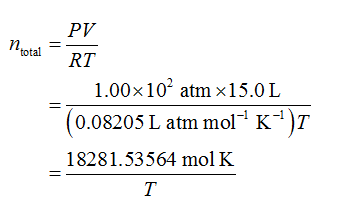It was given that tank cannot be emptied below 1.0 atm. Therefore, the number of moles of helium that is remain in the tank is calculated as shown below.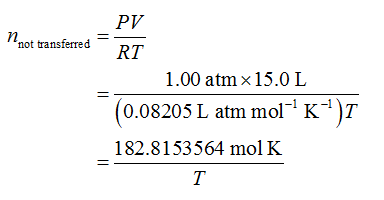Therefore, the number of moles of helium gas transferred from the tank to balloons is calculated as shown below.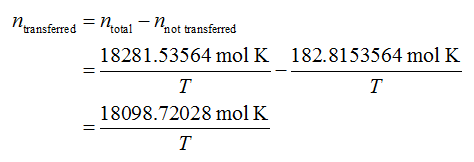Substitute the volume as 2.0 L and pressure as 1.00 atm in equation (I) to calculate the number of moles of helium gas in each balloon.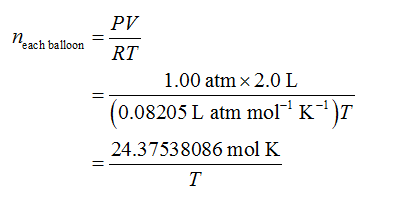The number of balloons is calculated as shown below.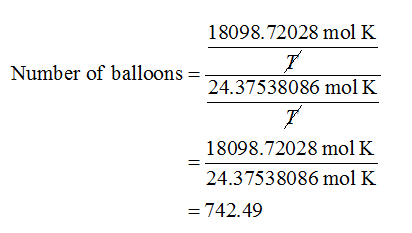Since the number of balloons can not be in fraction or in decimal. Therefore, the number of balloons will be 742.

...

### Want to see the full answer?

See Solution

#### Want to see this answer and more?

Solutions are written by subject experts who are available 24/7. Questions are typically answered within 1 hour.*

See Solution
*Response times may vary by subject and question.
Tagged in